# The Omnitruncated 120-cell

The omnitruncated 120-cell, also known as the omnitruncated 600-cell, is the largest member in the 120-cell/600-cell family of uniform polychora. It has 14400 vertices, 28800 edges, 17040 polygons (10800 squares, 4800 hexagons, and 1440 decagons), and 2640 cells (120 great rhombicosidodecahedra, 720 decagonal prisms, 1200 hexagonal prisms, and 600 truncated octahedra).

The omnitruncated 120-cell may be constructed by radially expanding the great rhombicosidodecahedral cells of the cantitruncated 120-cell outwards, and filling the gaps with decagonal prisms, hexagonal prisms, and truncated octahedra.

## Structure

We shall explore the structure of the omnitruncated 120-cell by its parallel projections into 3D, centered on one of its great rhombicosidodecahedral cells.

### First Layer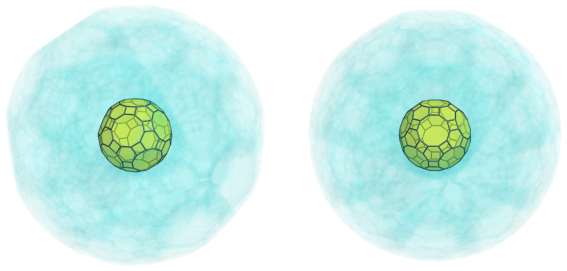The above image shows the nearest great rhombicosidodecahedron to the 4D viewpoint. For the sake of clarity, we have rendered all the other cells in a light transparent color.

The decagonal faces of this nearest cell are joined to 12 decagonal prisms, as shown in the next image: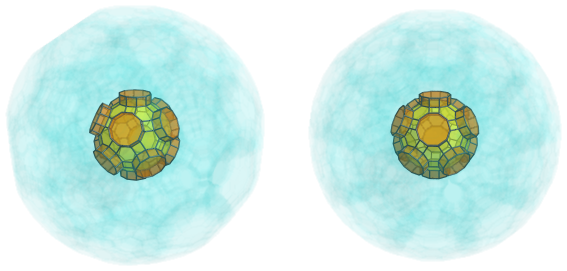The square faces of the nearest cell are joined to 30 hexagonal prisms, shown below in blue: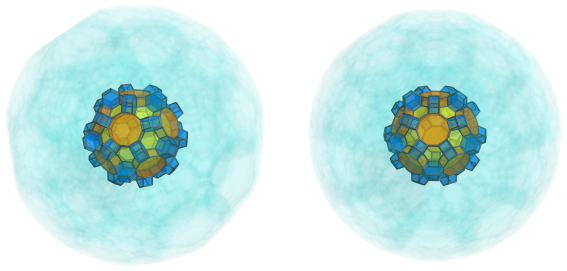Finally, the hexagonal faces of the nearest cell are joined to 20 truncated octahedra, shown next in green: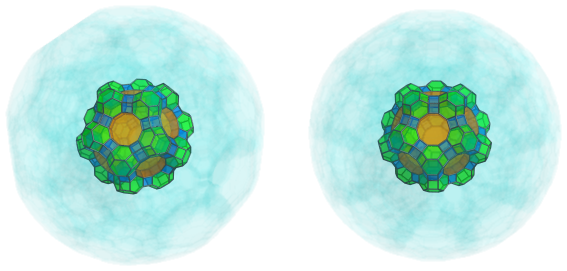### Second Layer

On top of the truncated octahedra from the previous layer are another 20 hexagonal prisms: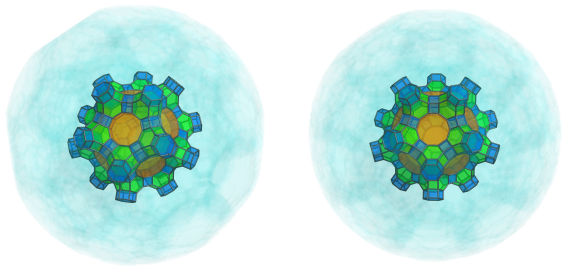Fitting into the valleys between these hexagonal prisms are 30 more decagonal prisms: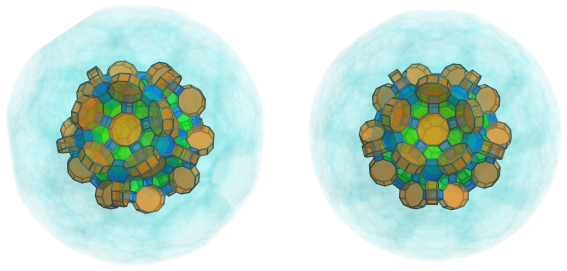Capping the hexagonal prisms and straddling these decagonal prisms are another 20 truncated octahedra: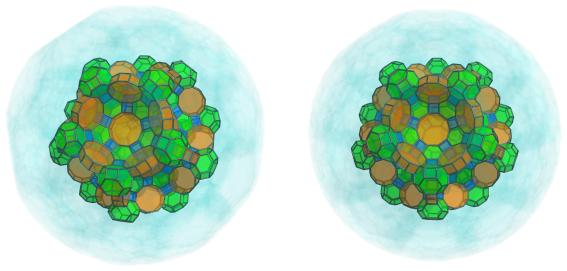The bowl-shaped depressions that are becoming obvious are the seats of 12 more great rhombicosidodecahedral cells: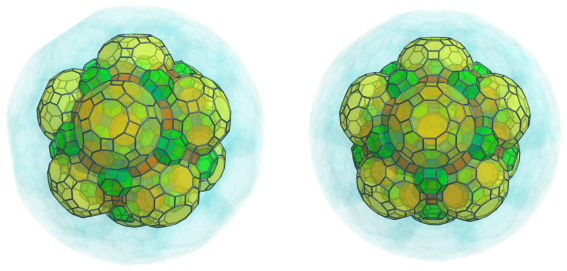### Third Layer

The pattern of alternating hexagonal prisms and truncated octahedra continues from the previous layer between the great rhombicosidodecahedral cells last seen. The following image shows 60 more hexagonal prisms: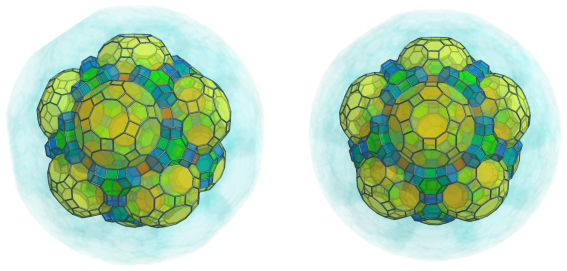These hexagonal prisms are capped by another 30 truncated octahedra: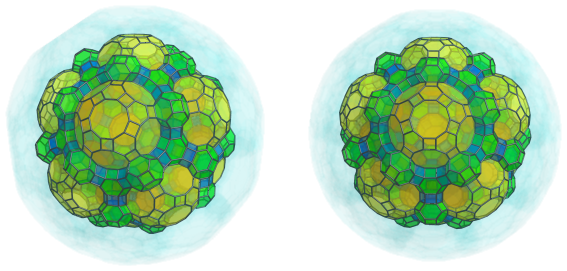The little inlets between these alternating cells are where 60 more decagonal prisms fit: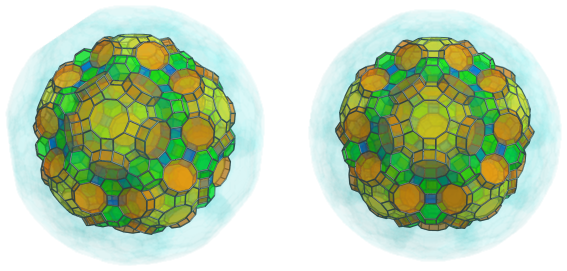The alternating cells actually encircle these decagonal prisms; for example, there are 60 more hexagonal prisms that fit between them: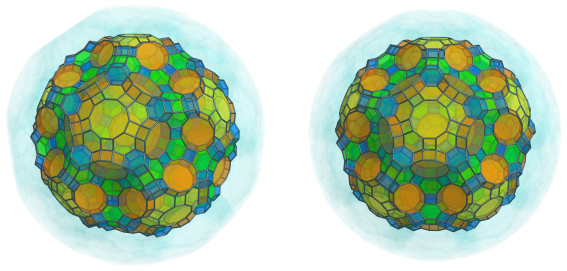The other side of these prisms are, of course, attached to more truncated octahedra, another 60 of them: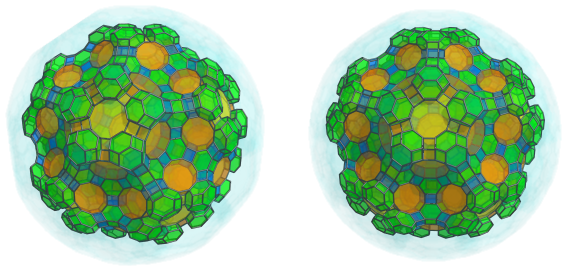These truncated octahedra converge on 12 more decagonal prisms: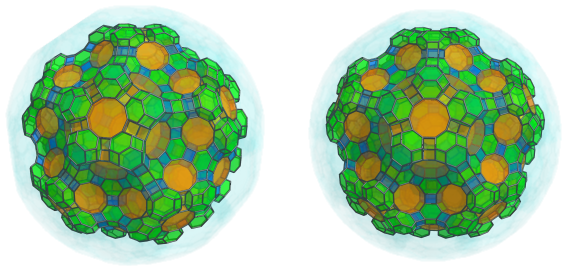The truncated octahedra are also bridged by 60 more hexagonal prisms: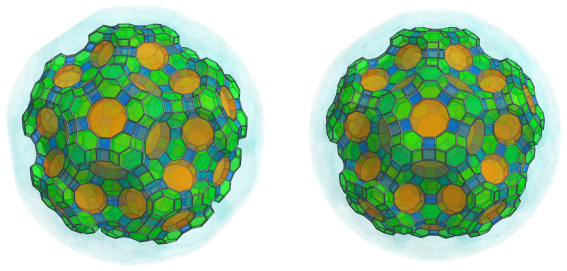The bowl-shaped depressions that are starting to form from these alternating cells are where 20 more great rhombicosidodecahedra are joined: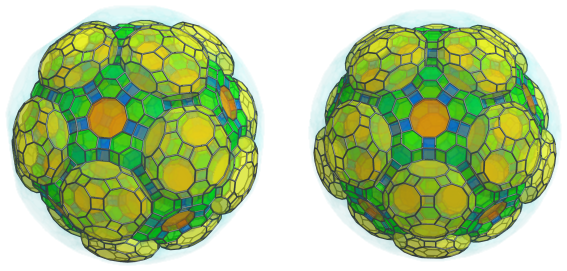#### Fourth Layer

The great rhombicosidodecahedra from the previous layer are linked to each other by 30 decagonal prisms: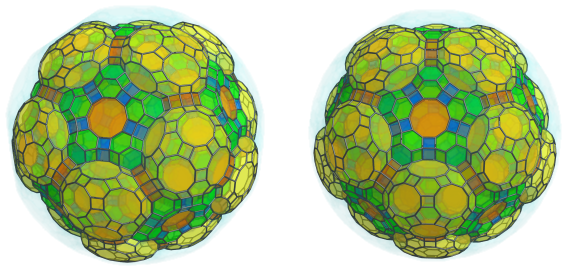On either side of these prisms, 60 more hexagonal prisms continue the pattern of alternating hexagonal prisms and truncated octahedra: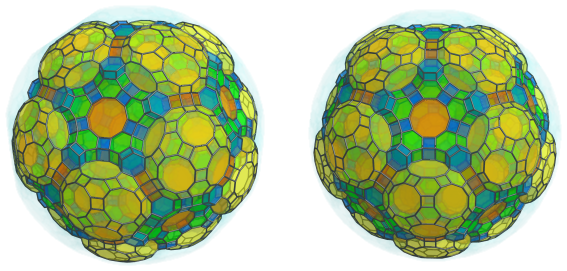It may not have been obvious before, but these hexagonal prisms also form an alternating pattern with the decagonal prisms, 60 more of which are shown below: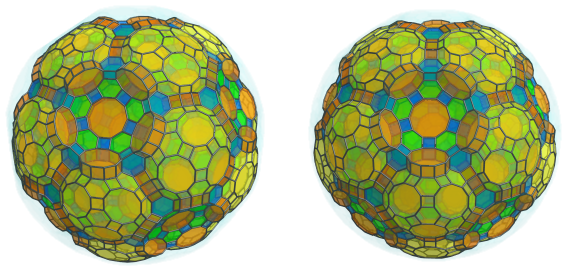The truncated octahedra also alternate with these decagonal prisms, and the three types of cells form an interlocking network. The next image shows 60 of these truncated octahedra in alternating formation with the decagonal prisms: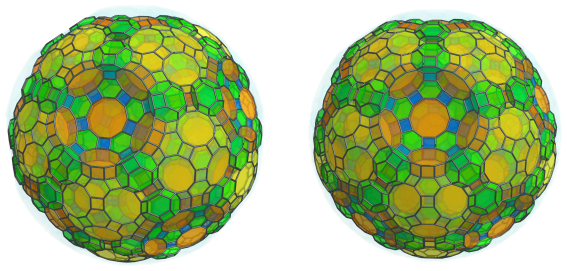These circles of truncated octahedra are linked to each other via 30 more hexagonal prisms: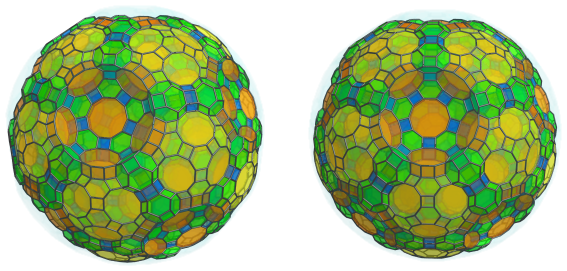The obvious bowl-shaped depressions are where another 12 great rhombicosidodecahedra are fitted: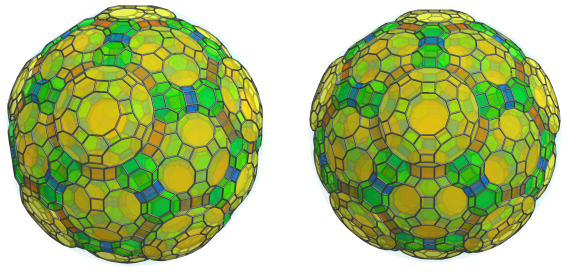### Fifth Layer

Of course, at the base of these great rhombicosidodecahedra there are also more hexagonal prisms emanating from the truncated octahedra within their five-fold circles, for a total of another 120 hexagonal prisms: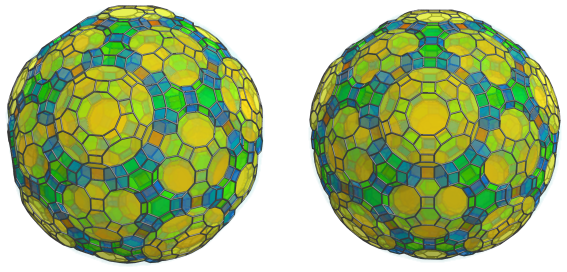These hexagonal prisms converge on another 60 truncated octahedra: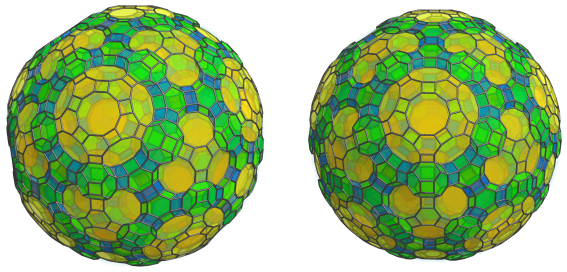Alternating with these truncated octahedra are another 60 decagonal prisms: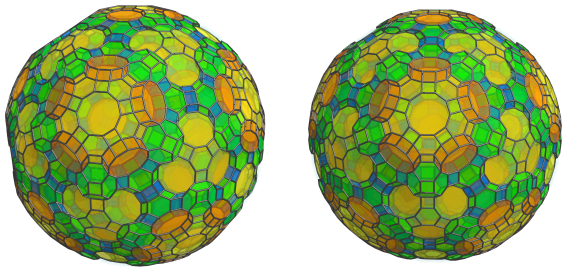These decagonal prisms, in turn, alternate with yet another 60 hexagonal prisms: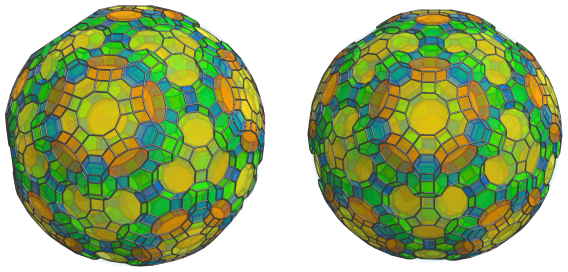On the other side of the truncated octahedra are some obvious gaps where 60 more decagonal prisms fit: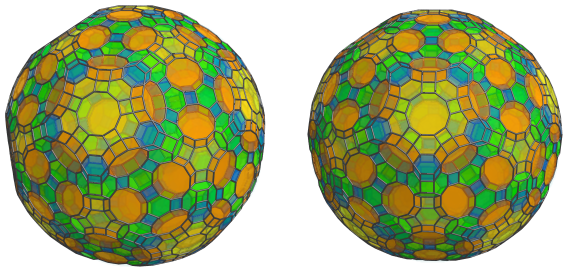Straddling these decagonal prisms and touching the truncated octahedra are another 60 hexagonal prisms, the last before we reach the “equator” of the omnitruncated 120-cell: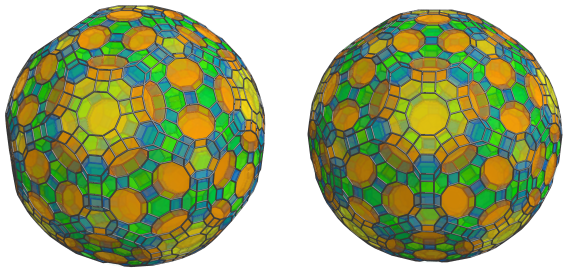Finally, these hexagonal prisms converge on 20 truncated octahedra: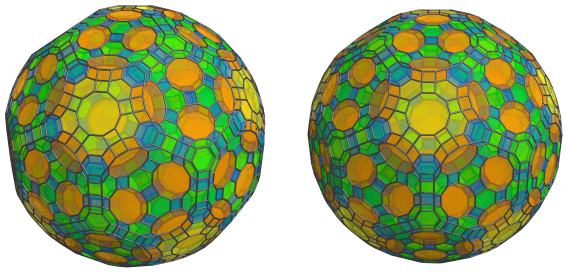These are all the cells that lie on the near side of the omnitruncated 120-cell. Past this point, we reach the limb, or “equator”, of the polytope.

### The Equator

Now we come to the equator of the omnitruncated 120-cell. There are 30 great rhombicosidodecahedra on the equator: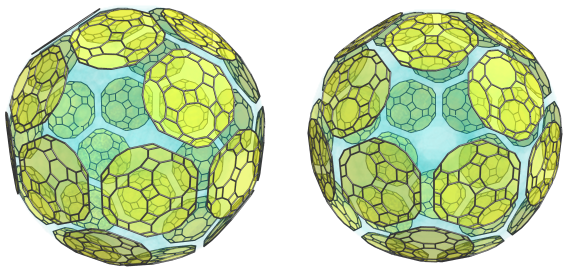For clarity, we have omitted the other cells that we have seen so far. These cells appear flattened into irregular dodecagons; this is because they are being seen at a 90° angle from the 4D viewpoint. In 4D, they are perfectly uniform great rhombicosidodecahedra.

There are 20 hexagonal prisms where each three of these cells meet: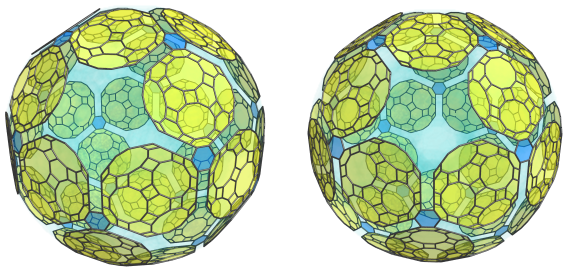These hexagonal prisms have been foreshortened into hexagons because of the 90° view angle.

They aren't the only hexagonal prisms on the equator; there are 60 others that touch the great rhombicosidodecahedra: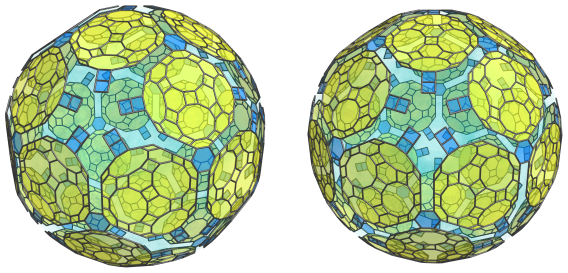These hexagonal prisms have a different orientation from the previous ones, hence they appear foreshortened into rectangles instead of hexagons. They alternate with 60 truncated octahedra: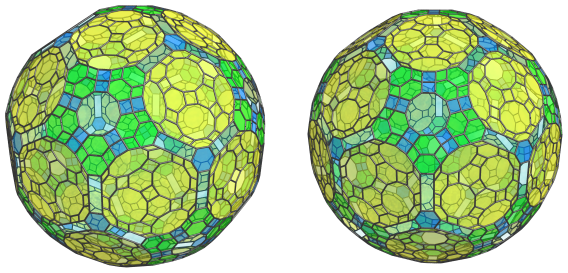As with the other equatorial cells, these truncated octahedra appear flattened into hexagons because they lie at a 90° angle to the 4D viewpoint. In 4D, they are perfectly uniform truncated octahedra.

These hexagonal prisms and truncated octahedra meet at 12 decagonal prisms: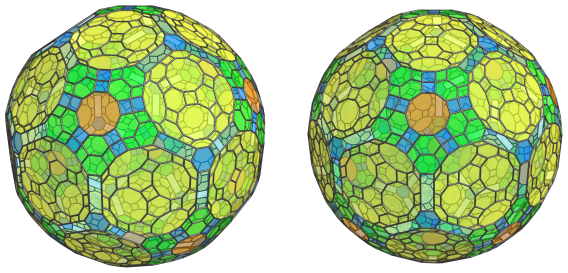These decagonal prisms appear foreshortened into decagons due to their 90° angle with the 4D viewpoint. They are not the only decagonal prisms on the equator; the remaining rectangular gaps are filled by another 60 decagonal prisms: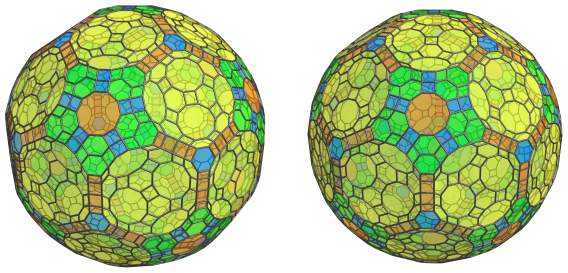These decagonal prisms are in a different orientation from the previous ones; hence, they appear foreshortened into rectangles instead of decagons.

These are all the cells that lie on the equator of the omnitruncated 120-cell. Past this point, we reach the far side of the polytope, where the arrangement of cells exactly mirrors that of the near side that we have seen, repeating in reverse order until we reach the antipodal great rhombicosidodecahedron.

### Summary

The following table shows the summary of the cell counts in each layer of the omnitruncated 120-cell:

Region Layer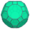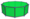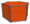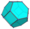Near side 1 1 12 30 20
2 12 30 20 20
3 20 60 + 12 = 72 60 + 60 + 60 = 180 30 + 60 = 90
4 12 30 + 60 = 90 60 + 30 = 90 60
5 0 60 + 60 = 120 120 + 60 + 60 = 240 60 + 20 = 80
Subtotal 45 324 560 270
Equator 30 12 + 60 = 72 20 + 60 = 80 60
Far side 5 0 120 240 80
4 12 90 90 60
3 20 72 180 90
2 12 30 20 20
1 1 12 30 20
Subtotal 45 324 560 270
Grand total 120 720 1200 600

## Coordinates

The coordinates of an origin-centered omnitruncated 120-cell with edge length 2 are all permutations of coordinates and changes of sign of:

 (1, 1, 1+6φ, 7+10φ) (1, 1, 3+8φ, 7+8φ) (1, 1, 1+4φ, 5+12φ) (1, 3, φ6, φ6) (2, 2, 4φ3, 6+8φ) (2φ2, 4+2φ, 4φ3, 4φ3) (3+2φ, 3+2φ, 3+8φ, φ6) (1+4φ, 3+4φ, 3+8φ, 3+8φ) (2φ3, 2φ3, 2+8φ, 4φ3)

along with all even permutations of coordinate and all changes of sign of:

 (1, 5φ2, 4+7φ, 6φ2) (1, 2φ4, 5+7φ, 6+5φ) (1, 2, 1+5φ, 6+11φ) (1, φ2, 6+9φ, 2+8φ) (1, φ2, 8+9φ, 2+6φ) (1, 2φ, 7+9φ, 2+7φ) (1, φ3, 5+12φ, 3+2φ) (1, 3+φ, 4+9φ, 4φ3) (1, 1+3φ, 8+9φ, 4φ2) (1, 1+3φ, 6+11φ, 4+2φ) (1, 4φ, 7+9φ, 4+5φ) (1, 3φ2, 4+9φ, 6φ2) (1, 2φ3, 5+9φ, 6+5φ) (2, φ2, 5+12φ, φ4) (2, 2+φ, 5+9φ, 3+8φ) (2, φ3, 8+9φ, φ5) (2, 3φ, 7+9φ, 3φ3) (2, 1+3φ, 7+10φ, 4+3φ) (2, 3+2φ, 4+9φ, 5+7φ) (2, 1+4φ, 6+9φ, 5φ2) (φ2, 4+5φ, 3+8φ, 6φ2) (φ2, 3φ3, 4φ3, 6+5φ) (φ2, 3, 2φ3, 6+11φ) (φ2, 3φ, 5+12φ, 2φ2) (φ2, 3+2φ, 4φ, 6+11φ) (φ2, 4+3φ, φ6, 6φ2) (φ2, 3+4φ, 6+8φ, 6+5φ) (3, φ3, 7+10φ, 3+4φ) (3, 2φ2, 5+9φ, 4+7φ) (3, 1+3φ, 6+9φ, 2φ4) (2φ, 4φ2, 4φ3, 6φ2) (2φ, φ5, φ6, 6+5φ) (2φ, 2+φ, 1+3φ, 5+12φ) (2φ, 3+φ, 1+4φ, 6+11φ) (2+φ, 4φ, 7+10φ, 3φ2) (2+φ, 4+2φ, φ6, 5+7φ) (2+φ, 2φ3, 7+8φ, 5φ2) (φ3, 4+5φ, 2+8φ, 5+7φ) (φ3, 5φ2, 2+7φ, 4φ3) (3+φ, 3φ, 7+10φ, 2φ3) (3+φ, 3+2φ, 6+8φ, 4+7φ) (3+φ, φ4, 7+8φ, 2φ4) (3φ, 4φ2, 3+8φ, 5+7φ) (3φ, 2+6φ, φ6, 5φ2) (2φ2, 1+4φ, 8+9φ, 3φ2) (2φ2, 1+5φ, 7+8φ, 4+5φ) (1+3φ, 1+6φ, 6+8φ, 4+5φ) (1+3φ, 3φ3, 2+8φ, 4+7φ) (1+3φ, 2+7φ, 3+8φ, 2φ4) (1+3φ, 3+2φ, 2φ3, 8+9φ) (1+3φ, 4+3φ, 3+8φ, 4φ3) (3+2φ, 1+4φ, 7+8φ, 3φ3) (3+2φ, 2φ3, 7+9φ, 4+3φ) (3+2φ, 1+5φ, 6+9φ, 4φ2) (4φ, φ5, 3+8φ, 4+7φ) (4φ, 2+6φ, 4φ3, 2φ4) (φ4, 4φ2, 1+6φ, 5+9φ) (φ4, 4+2φ, 3+4φ, 7+9φ) (φ4, 3φ2, 2+8φ, φ6) (4+2φ, 1+4φ, 6+9φ, φ5) (1+4φ, 1+6φ, φ6, 3φ3) (1+4φ, 3φ2, 2+7φ, 6+8φ) (1+4φ, 4+3φ, 2+6φ, 5+9φ) (2φ3, 1+6φ, 4+9φ, φ5) (2φ3, 1+5φ, φ6, 2+7φ) (1+5φ, 3+4φ, 2+6φ, 4+9φ)

where φ=(1+√5)/2 is the Golden Ratio.

Last updated 03 Sep 2018.